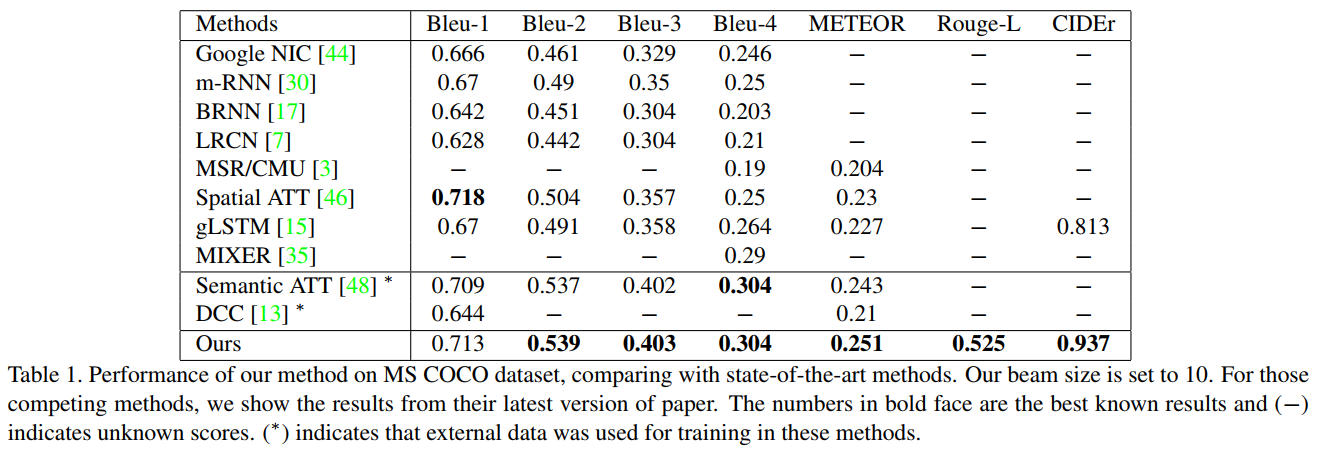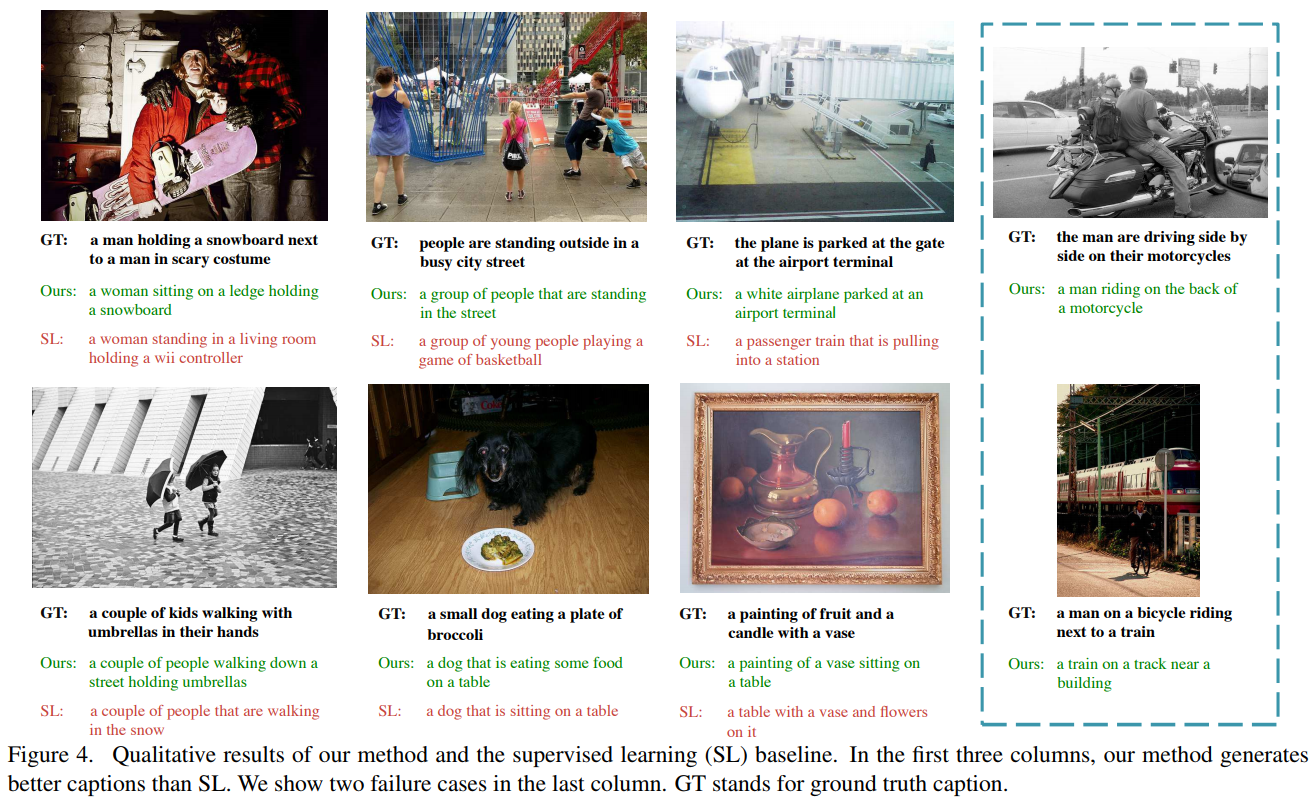The task of the model is to generate image captioning for the MSCOCO dataset using reinforcement learning. The novelty of this work is in two part :

• The decision making based on a policy $$p_\pi$$ and a value $$v_\theta$$ network.
• A training of $$p_\pi$$ and $$v_\theta$$ based on an actor-critic algorithm with a visual-semantic embedding reward.

## Model

Their model is separated in three parts, the policy network, the value network and the visual-semantic embedding.

#### Policy network

The policy is a combination of the VGG16 and an LSTM. It is used to predict the next action given the current state, as shown in figure 2.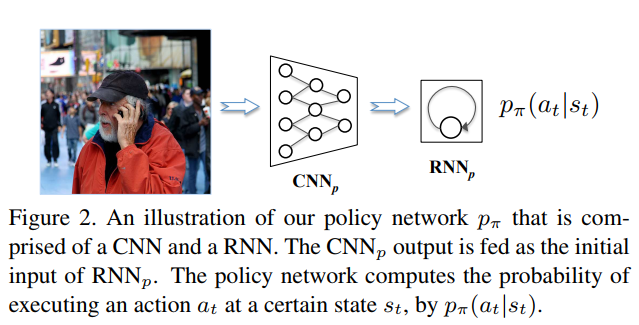#### Value network

The value network has the same combination as the policy network but they add an MLP used for the regression task. It is used to evaluate the next word given the image features and the previous words sentence generated in the sentence.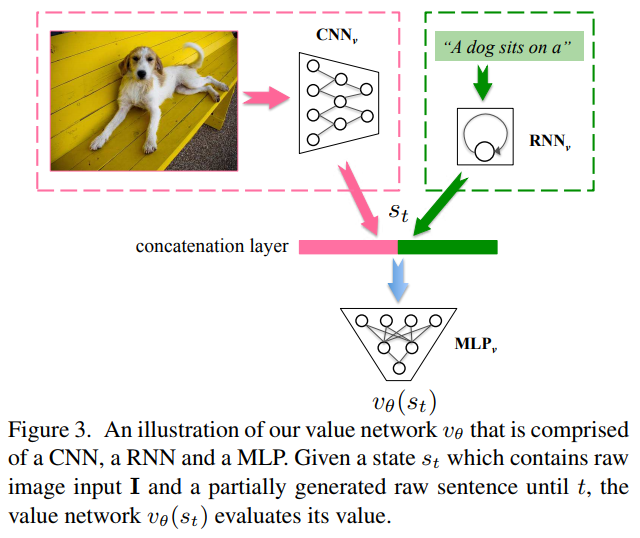#### Training

These two networks are first pretrained before using the reinforcement learning algorithm to make them work together. The policy network is trained using a crossentropy on the sentence, and the value network is trained to do a regression task on the final reward.

After this pretaining, they use the actor-critic algrotihm to train both of them, and use the visual-semantic embedding as a reward.

#### Visual-semantic embedding

The visual-semantic embedding is a model composed in three parts, a VGG16, a GRU, and a linear mapping layer. The embedding space is used to provide a similarity mesure between sentences and images.

They use a bidirectional ranking loss to train it, where $$v$$ is the image features vector, $$f_e(.)$$ is the mapping into the embedding space, and $$h'_T(.)$$ is the features of the last RNN state.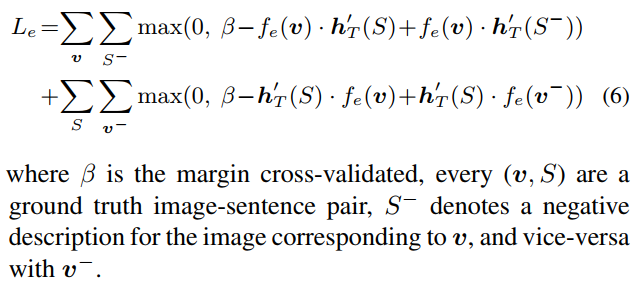## Inference

For the prediction they use the policy network as a local guidance and the value network as a global guidance. They use BeamSearch to select the top $$B$$ words to continue the sentence. The score for each word is given by,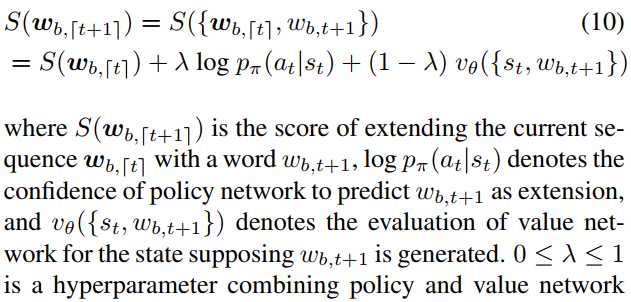The global picture of the inference is shown in figure 1.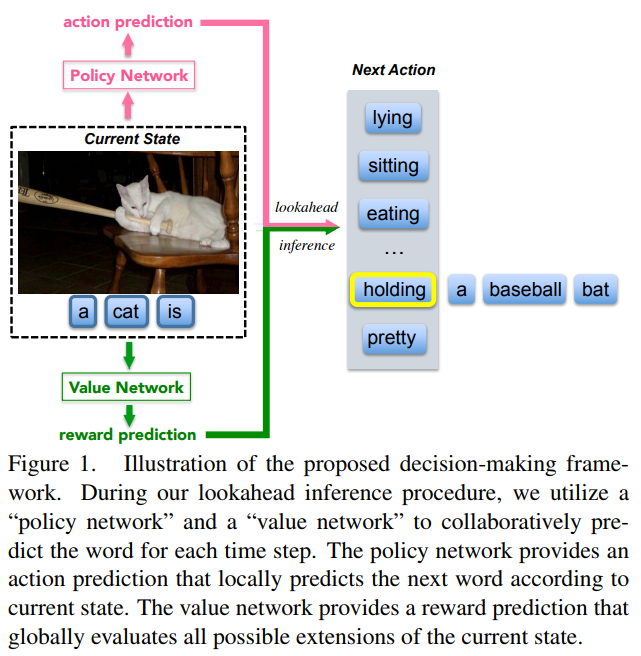## Results

They report results on MSCOCO caption dataset, using the BLEU metric.